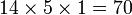# Groups of order 2160

## Contents

This article gives information about, and links to more details on, groups of order 2160
See pages on algebraic structures of order 2160| See pages on groups of a particular order

## Statistics at a glance

The number 2160 has the prime factorization:$\! 2160 = 2^4 \cdot 3^3 \cdot 5^1 = 16 \cdot 27 \cdot 5$

All groups of this order have not yet been classified. The information below is therefore partial.

Quantity Value Explanation
Total number of groups up to isomorphism 3429
Number of abelian groups (i.e., finite abelian groups) up to isomorphism 15 (Number of abelian groups of order$2^4$) times (Number of abelian groups of order$3^3$) times (Number of abelian groups of order$5^1$) = (number of unordered integer partitions of 4) times (number of unordered integer partitions of 3) times (number of unordered integer partitions of 1) =$5 \times 3 \times 1 = 15$. See classification of finite abelian groups and structure theorem for finitely generated abelian groups.
Number of nilpotent groups (i.e., finite nilpotent groups) up to isomorphism 70 (Number of groups of order 16) times (Number of groups of order 27) times (Number of groups of order 5) =$14 \times 5 \times 1 = 70$. See number of nilpotent groups equals product of number of groups of order each maximal prime power divisor, which in turn follows from equivalence of definitions of finite nilpotent group.
Number of solvable groups (i.e., finite solvable groups) up to isomorphism unknown, but likely between 1000 and 10000
Number of non-solvable groups up to isomorphism PLACEHOLDER FOR INFORMATION TO BE FILLED IN: [SHOW MORE] There are two possibilities for the composition factors of non-solvable groups:
First: alternating group:A5 (order 60) as the only simple non-abelian composition factor, cyclic group:Z2 (2 times), cyclic group:Z3 (2 times)
Second: alternating group:A6 (order 360) as the only simple non-abelian composition factor, cyclic group:Z2 (1 time), cyclic group:Z3 (1 time)
Number of simple groups up to isomorphism 0
Number of almost simple groups up to isomorphism 0
Number of quasisimple groups up to isomorphism 1 Schur cover of alternating group:A6